DraftKings 2019 Western Conference Odds
+600
6 to 1Avalanche
11.5% implied probability

+600
6 to 1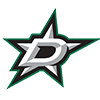Stars
11.5% implied probability

+600
6 to 1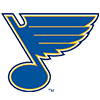Blues
11.5% implied probability

+700
7 to 1Golden Knights
10.0% implied probability

+750
7.5 to 1Predators
9.4% implied probability

+800
8 to 1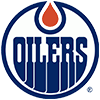Oilers
8.9% implied probability

+1000
10 to 1Coyotes
7.3% implied probability

+1150
11.5 to 1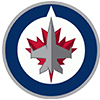Jets
6.4% implied probability

+1400
14 to 1Canucks
5.3% implied probability

+1500
15 to 1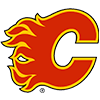Flames
5.0% implied probability

+1500
15 to 1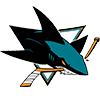Sharks
5.0% implied probability

+2700
27 to 1Wild
2.9% implied probability

+3000
30 to 1Ducks
2.6% implied probability

+4000
40 to 1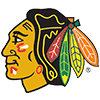Blackhawks
2.0% implied probability

+10000
100 to 1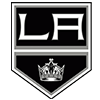Kings
0.8% implied probability